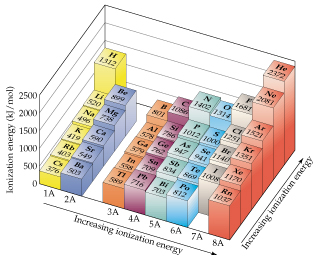# Problem: One way to measure ionization energies is photoelectron spectroscopy (PES), a technique based on the photoelectric effect. In PES, monochromatic light is directed onto a sample, causing electrons to be emitted. The kinetic energy of the emitted electrons is measured. The difference between the energy of the photons and the kinetic energy of the electrons corresponds to the energy needed to remove the electrons (that is, the ionization energy). Suppose that a PES experiment is performed in which mercury vapor is irradiated with ultraviolet light of wavelength 58.4 nm. What is the energy of a photon of this light, in eV?

###### FREE Expert Solution

We’re being asked to calculate the energy of a photon (eV) with a wavelength of 58.4 nm

Recall that the energy of a photon (E) is given by:

where:

h = Planck’s constant (6.626 × 10–34 J • s)

v = frequency (in s–1)

Also, recall that the frequency (v) and wavelength (λ) are related:

where:

c = speed of light (3.0 × 108 m/s)

79% (211 ratings)###### Problem Details

One way to measure ionization energies is photoelectron spectroscopy (PES), a technique based on the photoelectric effect. In PES, monochromatic light is directed onto a sample, causing electrons to be emitted. The kinetic energy of the emitted electrons is measured. The difference between the energy of the photons and the kinetic energy of the electrons corresponds to the energy needed to remove the electrons (that is, the ionization energy). Suppose that a PES experiment is performed in which mercury vapor is irradiated with ultraviolet light of wavelength 58.4 nm.What is the energy of a photon of this light, in eV?